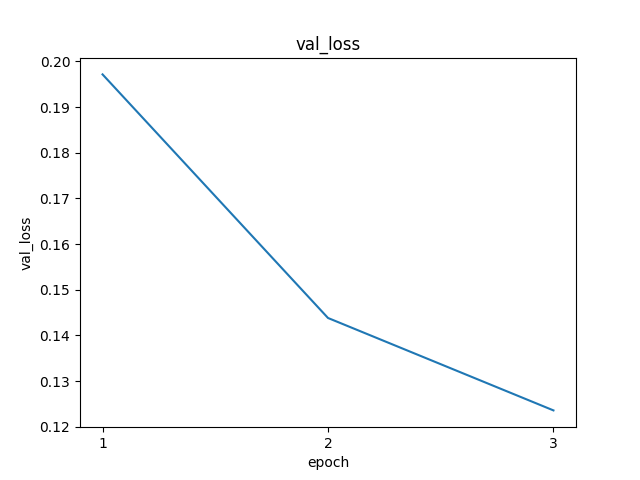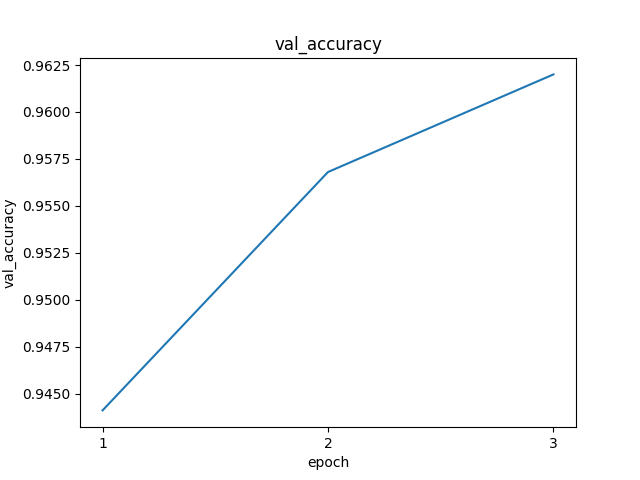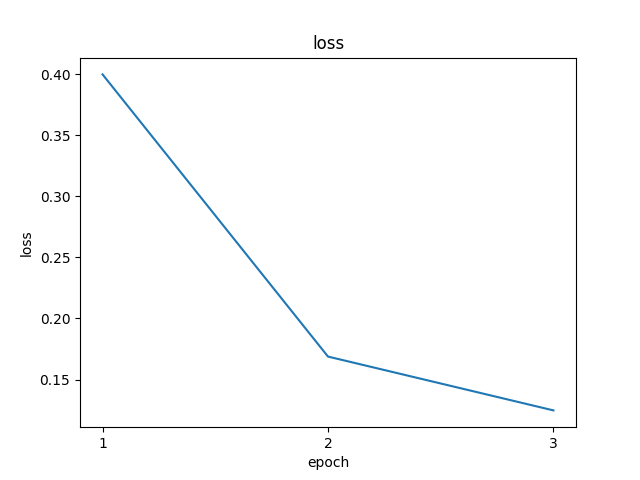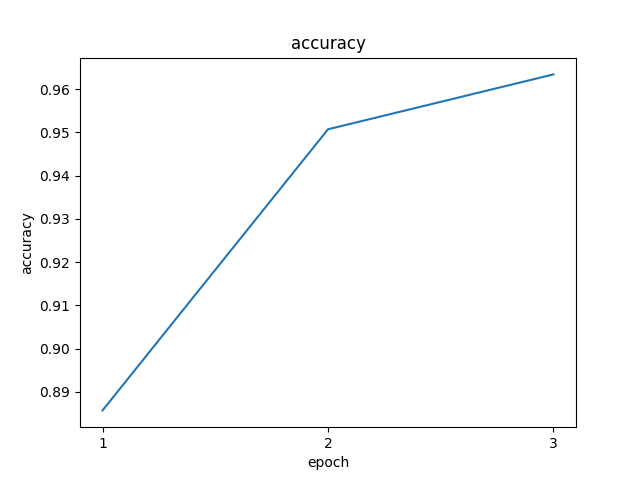# UbiOps Checkpoint TensorFlow¶

In this example, we train a simple model, to show how to save checkpoints in our file storage.
During the training run, we save model checkpoints to our file storage, making use of the TensorFlow callback class. At the end of our training run, we save plots of performance metrics.

First of all, let's install the required packages with pip in the current virtual environment!

``````!pip install "ubiops >= 3.15"
``````

Now it's time to set up all our project variables and to connect to our project using the UbiOps Client Library:

``````import ubiops

API_TOKEN = "Token ..." # Add an API Token with 'project editor' rights on your project

ENVIRONMENT_NAME = "checkpoint-tf-env"
EXPERIMENT_NAME = "checkpoint-tf-experiment"
``````
``````configuration = ubiops.Configuration(host="https://api.ubiops.com/v2.1")
configuration.api_key['Authorization'] = API_TOKEN

api_client = ubiops.ApiClient(configuration)
core_instance = ubiops.CoreApi(api_client=api_client)
training_instance = ubiops.Training(api_client=api_client)
print(core_instance.service_status())
``````

In this example, a very simple model is used to illustrate the checkpointing functionality.
We train a small Convolutional Neural network on the MNIST dataset. The training job will be run inside the UbiOps training section, so the model code will be wrapped into the UbiOps training function!

Let's create 2 different directories, one directory to save the environment code and another to save our training code!

``````!mkdir training_environment
!mkdir training_code
``````

All our pip packages should be specified in a requirements.txt file for our environment!

``````%%writefile training_environment/requirements.txt
ubiops >= 3.15
tensorflow
matplotlib
numpy
joblib
``````

Now, we want to create a `train.py` file where our training code will be stored. The code will be explained after the code is given!

``````%%writefile training_code/train.py
import os

import joblib
import matplotlib.pyplot as plt
import numpy as np
import tensorflow as tf
import ubiops

checkpoint_dir = "checkpoint"

project_name = "checkpoint-tensorflow"

class UbiOpsCallback(tf.keras.callbacks.Callback):
def __init__(self, bucket_name, context):
super().__init__()
self.bucket_name = bucket_name
self.global_logs = {}
self.client_prod = ubiops.ApiClient(
ubiops.Configuration(api_key={'Authorization': os.environ["UBIOPS_API_TOKEN"]})
)
self.context = context

def on_epoch_end(self, epoch, logs=None):
"""
This function is called at the end of each epoch. The function will upload the current model to UbiOps
for checkpointing.

:param epoch: the epoch number
:param logs: the logs of the epoch
"""

print("\nEpoch Finished: Logs are:", logs)

model_dir = 'model_checkpoint'
if not os.path.exists(model_dir):
os.makedirs(model_dir)

model_name = 'model'
joblib.dump(self.model, f"{model_dir}/{model_name}.joblib")

client=self.client_prod,
project_name=project_name,
file_path=f"{model_dir}/{model_name}.joblib",
bucket_name=self.bucket_name,
file_name=f"deployment_requests/{self.context['id']}/checkpoints/model_epoch_{epoch}.joblib"
)

# Update the global logs
self.global_logs.update({metric: self.global_logs.get(metric, []) + [value] for metric, value in logs.items()})

def on_train_end(self, logs=None):
print("Training Finished")
self.plot_logs()

def plot_logs(self):
"""
This function will plot the logs of the training and save them to the figure folder for later inspection.
"""

# Check if figure folder exists
if not os.path.exists("figure"):
os.makedirs("figure")

for key in self.global_logs:
file_name = f"figure/{key}.png"
plt.figure()
plt.title(key)

epochs = np.arange(1, len(self.global_logs[key]) + 1)
plt.plot(epochs, self.global_logs[key])
plt.ylabel(key)
plt.xlabel('epoch')
plt.xticks(np.arange(min(epochs), max(epochs) + 1, 1))
plt.savefig(file_name)
plt.show()
plt.close()

client=self.client_prod,
project_name=project_name,
file_path=file_name,
bucket_name=self.bucket_name,
)

def train(training_data, parameters, context):
print(f"Training data: {training_data}")
print(f"Parameters: {parameters}")
print(f"Context: {context}")

# Define the model architecture
model = tf.keras.Sequential([
tf.keras.layers.Dense(64, activation='relu', input_shape=(784,)),
tf.keras.layers.Dense(64, activation='relu'),
tf.keras.layers.Dense(10, activation='softmax')
])

# Compile the model
loss='categorical_crossentropy',
metrics=['accuracy'])

# Set callback
custom_callback = UbiOpsCallback(bucket_name="default", context=context)

# Load data and train the model
(x_train, y_train), (x_test, y_test) = tf.keras.datasets.mnist.load_data()
x_train = x_train.reshape((60000, 784)).astype('float32') / 255.0
x_test = x_test.reshape((10000, 784)).astype('float32') / 255.0
y_train = tf.keras.utils.to_categorical(y_train)
y_test = tf.keras.utils.to_categorical(y_test)

epochs = parameters.get("epochs", 3)
batch_size = parameters.get("batch_size", 128)

result = model.fit(x_train, y_train, epochs=epochs, batch_size=batch_size, validation_data=(x_test, y_test),
callbacks=[custom_callback])

# Get the loss and accuracy
loss, accuracy = model.evaluate(x_test, y_test)

# Save the model
joblib.dump(model, "model.joblib")

return {
"artifact": "model.joblib",
"metrics": {"accuracy": accuracy},
}
``````

As seen in the code above, the checkpointing is done by specifying a custom callback class `UbiOpsCallback` and setting that class as a callback in the `model.fit(...)` function.
After every epoch, the model in its current state will be saved in a bucket.
When the training is finished, the logs will be plotted in a graph and saved to a bucket to visually see how the model progressed after every epoch. Feel free to modify the code to your own liking, as this is just an example!

Let's zip the environment directory!

``````import shutil
training_environment_archive = shutil.make_archive('training_environment', 'zip', '.', 'training_environment')
``````

Let's enable the `training` functionality inside our project and create the environment!

``````try:
training_instance.initialize(project_name=PROJECT_NAME)
except ubiops.exceptions.ApiException as e:
print(f"The training feature may already have been initialized in your project: {e}")
``````
``````try:
core_instance.environments_create(
project_name=PROJECT_NAME,
data=ubiops.EnvironmentCreate(
name=ENVIRONMENT_NAME,
display_name=ENVIRONMENT_NAME,
base_environment='python3-10',
description='Ubiops checkpointing environment with TensorFlow',
)
)
except ubiops.exceptions.ApiException as e:
print(e)
``````
``````core_instance.environment_revisions_file_upload(
project_name=PROJECT_NAME,
environment_name=ENVIRONMENT_NAME,
file=training_environment_archive
)
``````

Let's wait for the environment to succeed!

``````ubiops.utils.wait_for_environment(core_instance.api_client, PROJECT_NAME, ENVIRONMENT_NAME, 600)
``````

Let's create an experiment now!

``````try:
experiment = training_instance.experiments_create(
project_name=PROJECT_NAME,
data=ubiops.ExperimentCreate(
instance_type='2048mb',
description='TensorFlow checkpointing experiment with UbiOps',
name=EXPERIMENT_NAME,
environment=ENVIRONMENT_NAME,
default_bucket='default'
)
)
except ubiops.exceptions.ApiException as e:
print(e)
``````

It's time to set our API Token as an environment variable. This way we can authenticate ourselves to upload files to a bucket, during our training run.

``````api_token_env_var = ubiops.EnvironmentVariableCreate(
name="UBIOPS_API_TOKEN",
value=API_TOKEN,
secret=True
)

core_instance.deployment_version_environment_variables_create(
project_name=PROJECT_NAME,
deployment_name="training-base-deployment",
version=EXPERIMENT_NAME,
data=api_token_env_var
)
``````

Now it's time to upload the training code!

``````from datetime import datetime
try:
new_run = training_instance.experiment_runs_create(
project_name=PROJECT_NAME,
experiment_name=EXPERIMENT_NAME,
data=ubiops.ExperimentRunCreate(
name=f"checkpoint-run-{datetime.now().isoformat()}",
description='checkpointing run',
training_code="training_code/train.py",
parameters=None,
timeout=14400
)
)
except ubiops.exceptions.ApiException as e:
print(e)
``````

After our experiment is finished, we can take a look (in the web app) at the different generated files! If we take a look at the folder that is created with our deployment request (easily found by clicking on the output artifact location in our exeriment results!), we can see the following 3 folders: - checkpoints - folder containing all our checkpoint models - figures - folder containing all our log figures - output - folder containing the final model

The following figures are created: